https://doi.org/10.5194/gmd-15-1995-2022
https://doi.org/10.5194/gmd-15-1995-2022# Empirical Lagrangian parametrization for wind-driven mixing of buoyant particles at the ocean surface

Victor Onink, Erik van Sebille, and Charlotte Laufkötter
Abstract

Turbulent mixing is a vital component of vertical particulate transport, but ocean global circulation models (OGCMs) generally have low-resolution representations of near-surface mixing. Furthermore, turbulence data are often not provided in OGCM model output. We present 1D parametrizations of wind-driven turbulent mixing in the ocean surface mixed layer that are designed to be easily included in 3D Lagrangian model experiments. Stochastic transport is computed by Markov-0 or Markov-1 models, and we discuss the advantages and disadvantages of two vertical profiles for the vertical diffusion coefficient Kz. All vertical diffusion profiles and stochastic transport models lead to stable concentration profiles for buoyant particles, which for particles with rise velocities of 0.03 and 0.003 m s−1 agree relatively well with concentration profiles from field measurements of microplastics when Langmuir-circulation-driven turbulence is accounted for. Markov-0 models provide good model performance for integration time steps of Δt≈30 s and can be readily applied when studying the behavior of buoyant particulates in the ocean. Markov-1 models do not consistently improve model performance relative to Markov-0 models and require an additional parameter that is poorly constrained.

Share
Dates
1 Introduction

Lagrangian models are essential tools to examine the transport of particulates in the ocean on a variety of spatial and temporal scales and have been used to study the movement of plastic particulates , oil and fish larvae . However, especially in the field of marine plastic modeling, most large-scale modeling studies consider only virtual particles (henceforth referred to as particles) that float and remain at the ocean surface , essentially simplifying the three-dimensional ocean into a 2D system. While this does reduce the complexity of models, ultimately vertical transport processes need to be considered in order to have a complete understanding of oceanic particulate transport .

In the case of buoyant particulates (particulates with a density lower than seawater), buoyancy is expected to return any particulates to the ocean surface. However, instead of all buoyant particulates accumulating at the ocean surface, both field measurements and regional large-eddy simulation (LES) model studies (e.g., ) indicate vertical concentration profiles throughout the mixed layer (ML). These profiles arise due to the balance between the particulate buoyancy and turbulent mixing flows, which are largely driven by wind and wave breaking at the ocean surface . While such profiles are commonly used to correct surface measurements of particulates such as microplastics (e.g., ), it is difficult to recreate such vertical mixing profiles in the ML outside of LES models, as vertical turbulent processes generally act on much smaller scales than is explicitly resolved in ocean global circulation models (OGCMs) (Taylor2018). In addition, while it is possible to represent mixing using the parametrization from , this approach is only valid for depths up to several meters, while the mixed-layer depth (MLD) can be hundreds of meters deep .

In this study we present numerical simulations of buoyant virtual particles in the ML with four 1D wind-driven mixing parametrizations. These mixing parametrizations have been specifically designed such that the code can be easily adapted to function within large-scale 3D Lagrangian models running with OGCM data, for cases where the vertical spatial scales might be too coarse to explicitly represent turbulent processes or where turbulence data might not be provided as model output. Using these parametrizations we calculate the vertical equilibrium profiles of buoyant particles within the ML as a function of the particle rise velocities, the 10 m wind speed and the MLD. Buoyant particles are found below the ML , but diffusive mixing at such depths is likely not due to wind-driven turbulent mixing and therefore goes beyond the scope of this study. We test two methods for solving stochastic differential equations and consider vertical diffusion coefficient profiles based on the K-profile parameterization (KPP) model and , which was extended by . The modeled concentration profiles are then compared with measurements of vertical concentration profiles of microplastics.

2 Model framework

## 2.1 Lagrangian stochastic transport

Turbulence in the ocean occurs over a wide range of spatial and temporal scales, with Kolmogorov length and timescales of $\mathit{\eta }=\left({\mathit{\nu }}^{\mathrm{3}}/\mathit{ϵ}{\right)}^{\mathrm{1}/\mathrm{4}}=\mathrm{3}×{\mathrm{10}}^{-\mathrm{4}}$ m and ${\mathit{\tau }}_{n}=\left(\mathit{\nu }/\mathit{ϵ}{\right)}^{\mathrm{1}/\mathrm{2}}=\mathrm{0.1}$ s for turbulent kinetic energy $\mathit{ϵ}={\mathrm{10}}^{-\mathrm{4}}$ m2 s−2 and kinematic viscosity of seawater $\mathit{\nu }={\mathrm{10}}^{-\mathrm{6}}$ m2 s−1 . The vertical resolution of OGCMs is typically on the order of meters and is therefore not capable of explicitly resolving all turbulent processes. Instead, turbulence due to sub-grid-scale processes is generally represented stochastically. In our 1D vertical model, we simulate positively buoyant particles that are vertically transported due to stochastic turbulence and the particle rise velocity wrise. For such particles, the particle trajectory Z(t) can be computed with a stochastic differential equation (SDE) as follows:

$\begin{array}{}\text{(1)}& Z\left(t+\mathrm{d}t\right)=Z\left(t\right)+\left({w}_{\mathrm{rise}}+{\partial }_{z}{K}_{z}\right)\mathrm{d}t+\sqrt{\mathrm{2}{K}_{z}}\mathrm{d}W,\text{(2)}& Z\left(\mathrm{0}\right)=\mathrm{0},\end{array}$

where Kz=Kz(Z(t)) is the vertical diffusion coefficient and ${\partial }_{z}{K}_{z}=\partial {K}_{z}/\partial z$, dW​​​​​​​ is a Wiener increment with zero mean and variance dt, and we define the vertical axis z as positive upward with z=0 at the air–sea interface. The Euler–Maruyama (EM) scheme (Maruyama1955) is the simplest numerical approximation of Eq. (1), where infinitesimal terms dt and dW are replaced with the finite terms Δt and ΔW. Equation (1) can then be rewritten as follows :

$\begin{array}{}\text{(3)}& {w}^{\prime }\left(t\right)={\partial }_{z}{K}_{z}+\frac{\mathrm{1}}{\mathrm{\Delta }t}\sqrt{\mathrm{2}{K}_{z}}\mathrm{\Delta }W,\text{(4)}& Z\left(t+\mathrm{\Delta }t\right)=Z\left(t\right)+\left({w}_{\mathrm{rise}}+{w}^{\prime }\left(t\right)\right)\mathrm{\Delta }t,\end{array}$

where w is the stochastic velocity perturbation due to turbulence. The turbulent transport has both a deterministic drift term and a stochastic term. This is the most basic form of representing turbulent particle transport, as turbulent perturbations on the particle position are assumed to be uncorrelated . The drift term assures that the well-mixed condition is met, which states that an initially uniform particle distribution must remain uniform even with inhomogeneous turbulence . This approach, termed a Markov-0 (M-0) or random walk model, assumes that turbulent fluctuations exhibit no autocorrelation on timescales Δt, which for global-scale Lagrangian simulations can range from 30 s to 30 min . However, measurements from Lagrangian ocean floats show this is an oversimplification, as coherent oceanic flow structures can induce velocity autocorrelations that can persist for significantly longer timescales .

A higher-order approach is the Markov-1 (M-1) model, which assumes a degree of autocorrelation of particle velocities set by the Lagrangian integral timescale TL. The turbulent velocity perturbation is now expressed as a Langevin equation, and with an EM numerical scheme the particle trajectory Z(t) is computed as follows :

$\begin{array}{}\text{(5)}& Z\left(t+\mathrm{\Delta }t\right)=Z\left(t\right)+\left({w}_{\mathrm{rise}}+{w}^{\prime }\left(t\right)\right)\mathrm{\Delta }t,\text{(6)}& {w}^{\prime }\left(t+\mathrm{\Delta }t\right)=\mathit{\alpha }{w}^{\prime }\left(t\right)+{\partial }_{z}{\mathit{\sigma }}_{w}^{\mathrm{2}}\mathrm{\Delta }t+\sqrt{\frac{\mathrm{2}\left(\mathrm{1}-\mathit{\alpha }\right){\mathit{\sigma }}_{w}^{\mathrm{2}}}{\mathrm{\Delta }t}}\mathrm{\Delta }W,\end{array}$

where $\mathit{\alpha }=\mathrm{1}-\mathrm{\Delta }t/{T}_{\mathrm{L}}$ and ${\mathit{\sigma }}_{w}^{\mathrm{2}}={\mathit{\sigma }}_{w}^{\mathrm{2}}\left(z,t\right)$ is the variance of w, and we assume ΔtTL. The influence of the initial turbulent fluctuations on subsequent fluctuations is set by α, which in turn depends on the ratio between the integration time step Δt and TL. However, empirical and theoretical estimates for TL range from 6–7 s to 15–30 min , and TL can also be depth dependent . In large-eddy simulation (LES) models, ${T}_{\mathrm{L}}=\mathrm{4}e/\mathrm{3}{C}_{\mathrm{0}}\mathit{ϵ}$, where e is the sub-grid scale turbulent kinetic energy, C0 is a model constant determining diffusion in the velocity space and ϵ is the turbulent kinetic energy dissipation rate , but e and ϵ are not commonly available variables in the output of OGCMs. However, it does indicate why model TL estimates vary widely, as TL describes the autocorrelation of the particle velocity from its initial velocity due to unresolved sub-grid processes, which depends on the model resolution and setup in a given study. Since there is not a clear indication of the true value of TL, we consider a range of values $\mathit{\alpha }\in \left[\mathrm{0},\mathrm{0.1},\mathrm{0.3},\mathrm{0.5},\mathrm{0.7},\mathrm{0.95}\right]$, corresponding to ${T}_{\mathrm{L}}\in \left[\mathrm{1},\mathrm{1.1},\mathrm{1.4},\mathrm{2},\mathrm{3.3},\mathrm{20}\right]×\mathrm{\Delta }t$. As the depth dependence of TL is uncertain, we make the simplification that ${\partial }_{z}{T}_{\mathrm{L}}={\partial }_{z}\mathit{\alpha }=\mathrm{0}$. Since ΔtTL, we use ${K}_{z}={\mathit{\sigma }}_{w}^{\mathrm{2}}\mathrm{\Delta }t$ , which means that Eq. (6) becomes

$\begin{array}{}\text{(7)}& {w}^{\prime }\left(t\right)=\mathit{\alpha }{w}^{\prime }\left(t\right)+{\partial }_{z}{K}_{z}+\frac{\mathrm{1}}{\mathrm{d}t}\sqrt{\mathrm{2}\left(\mathrm{1}-\mathit{\alpha }\right){K}_{z}}\mathrm{\Delta }W.\end{array}$

In this form, it is clear that Eq. (7) is equivalent to Eq. (4) when α=0. This is because when α=0, velocity perturbations w are assumed to be uncorrelated over timescales ≥Δt, which is equivalent to the M-0 formulation. M-1 stochastic models generally should lead to improved representation of diffusion in Lagrangian models , but it does require insight into turbulence statistics that have not yet been extensively studied in Lagrangian settings. For that reason, while even higher order Markov models are theoretically possible , we limit this study to just the M-0 and M-1 approaches.

All Lagrangian simulations are run using Parcels v2.2.1 , which has been used for 1D, 2D and 3D particle oceanographic simulations . The simulations start with 100 000 particles released at Z(0)=0 and run for 12 h. The model is one dimensional with horizontal velocities set to zero. The time-invariant vertical diffusion profiles are calculated with a 0.1 m vertical resolution, where the Kz value at the exact particle location is linearly interpolated from these profiles. The vertical transport is calculated according to Eqs. (3) and (4) for M-0 simulations and Eqs. (5) and (7) for M-1 simulations. We take Δt=30 s, where the integration time step is a compromise between accounting for turbulent transport on short timescales and computational cost for when the 1D model is integrated into a larger 3D Lagrangian model. We consider high, medium and low buoyancy particles with rise velocities of ${w}_{\mathrm{rise}}\in \left[\mathrm{0.03},\mathrm{0.003},\mathrm{0.0003}\right]$ m s−1, which for plastic polyethylene (ρ=980 kg m−3) particles corresponds to spherical particles with diameters of 2.2, 0.4 and 0.1 mm . However, these particle sizes are rough indications of approximate particle sizes, as the buoyancy of particle depends on a combination of the particle size, shape, polymer density and degree of biofouling . Relative to peak stochastic velocity perturbations w calculated from the vertical diffusion coefficients described in Sect. 2.2, the rise velocity of the high-buoyancy particles dominate w except for the highest wind speeds, while turbulence dominates buoyancy for the medium- and low-buoyancy particles for almost all wind conditions (Table A1). The surface wind stress is computed from ${u}_{\mathrm{10}}\in \left[\mathrm{0.85},\mathrm{2.4},\mathrm{4.35},\mathrm{6.65},\mathrm{9.3}\right]$ m s−1. The model domain is $z\in \left[-\mathrm{100},\mathrm{0}\right]$ m, where we apply a ceiling boundary condition (BC) in which particles that cross the surface boundary are placed at z=0. This BC assures that neither buoyancy or turbulence can transport particles out of the water column. Vertical concentration profiles are computed by binning the final particle locations into 0.5 m bins, and the concentrations are then normalized by the total number of particles in the simulation. The variability of the profiles at each depth level is calculated as the standard deviation over the final hour of each simulation.

## 2.2 Vertical diffusion profiles

Two vertical diffusion coefficient profiles are used, with the first based on and . parameterized the near-surface vertical diffusion coefficient ${K}_{z}^{\mathrm{S}}$ due to breaking waves as follows:

$\begin{array}{}\text{(8)}& {K}_{z}^{\mathrm{S}}=\mathrm{1.5}{u}_{*\mathrm{w}}\mathit{\kappa }{H}_{\mathrm{s}},\end{array}$

for $z>-\mathrm{1.5}{H}_{\mathrm{s}}$, where κ=0.4 is the von Karman constant, Hs is the significant wave height and u*w is the friction velocity of water. The significant wave height Hs is parameterized as ${H}_{\mathrm{s}}=\mathrm{0.96}{g}^{-\mathrm{1}}{\mathit{\beta }}_{*}^{\mathrm{3}/\mathrm{2}}{u}_{*\mathrm{a}}^{\mathrm{2}}$, where g=9.81 m s−2 is the acceleration of gravity, ${\mathit{\beta }}_{*}={c}_{\mathrm{p}}/{u}_{*\mathrm{a}}$ is the wave age (cp being the characteristic phase speed of the surface waves) and ${u}_{*\mathrm{a}}=\mathit{\tau }/{\mathit{\rho }}_{\mathrm{a}}$ is the friction velocity of water. The friction velocity of air is based on the air density ρa=1.22 kg m−3 and the surface wind stress $\mathit{\tau }={C}_{\mathrm{D}}{\mathit{\rho }}_{\mathrm{a}}{u}_{\mathrm{10}}^{\mathrm{2}}$, where u10 is the 10 m wind speed and CD is the drag coefficient . Similarly, ${u}_{*\mathrm{w}}=\mathit{\tau }/{\mathit{\rho }}_{\mathrm{w}}$ with the seawater density ρw=1027 kg m−3. Following , we assume a fully developed sea state with ${\mathit{\beta }}_{*}=\mathrm{35}$. The parametrization is valid only for $z\approx -\mathrm{1.5}{H}_{\mathrm{s}}$, and we extend the parametrization for greater depths using the eddy viscosity profile νz, as found for oscillating grid turbulence by :

where νS is the near-surface eddy viscosity and γ=1.0 is a multiple of Hs that sets the depth to which νS is constant. This approach agrees with in predicting constant mixing for $z>-{H}_{\mathrm{s}}$, where the eddy viscosity then drops proportional to ${z}^{-\mathrm{3}/\mathrm{2}}$ for greater depths. Oscillating grid turbulence (OGT) experiments are commonly used to study wave- and wind-induced turbulence (Fernando1991). As OGT experiments have been shown to reproduce turbulence decay laws of velocities and dissipation rates observed in the ocean ML , this provides some confidence in the modeling of the decay of near-surface eddy viscosity, although direct validation with field measurements of eddy viscosity have yet to occur. The diffusion coefficient Kz depends on νz as ${K}_{z}={\mathit{\nu }}_{z}/{\mathrm{Sc}}_{\mathrm{t}}$, where Sct is the turbulent Schmidt number, and assuming ${\partial }_{z}{\mathrm{Sc}}_{\mathrm{t}}=\mathrm{0}$, combining Eqs. (8) and (9) results in

where ${K}_{\mathrm{B}}=\mathrm{3}×{\mathrm{10}}^{-\mathrm{5}}$ m2 s−1 is the dianeutral diffusion below the MLD . The diffusion is thus constant for $z>-\mathit{\gamma }{H}_{\mathrm{s}}$, below which ${K}_{z}\propto |z{|}^{-\mathrm{3}/\mathrm{2}}$, while the magnitude of Kz increases for higher wind speeds (Fig. 1). implies γ=1.0, while estimates γ≈1.5, and thus to test the model sensitivity we consider $\mathit{\gamma }\in \left[\mathrm{0.5},\mathrm{1.0},\mathrm{1.5},\mathrm{2.0}\right]$ (Fig. 1). As $z\to -\mathrm{\infty }$, $|z{|}^{-\mathrm{3}/\mathrm{2}}\to \mathrm{0}$, and therefore we include the bulk dianeutral diffusion KB to account for vertical mixing at depths below the influence of surface wave-driven turbulence. As both and considered turbulence generated by breaking surface waves, we refer to this diffusion approach as surface wave breaking (SWB) diffusion.

The second vertical diffusion coefficient profile is a local form of the KPP , where Kz is given by

$\begin{array}{}\text{(11)}& {K}_{z}=\left(\frac{\mathit{\kappa }{u}_{*\mathrm{w}}}{\mathit{\varphi }}\mathit{\theta }\right)\left(|z|+{z}_{\mathrm{0}}\right)\left(\mathrm{1}-\frac{|z|}{\mathrm{MLD}}\right)+{K}_{\mathrm{B}},\end{array}$

where ϕ=0.9 is the “stability function” of the Monin–Obukhov boundary layer theory, θ is a Langmuir circulation (LC) enhancement factor and z0 is the roughness scale of turbulence. As such, Kz​​​​​​​ rises from a small non-zero value at z=0 to a maxima at $z=\mathrm{1}/\mathrm{3}\mathrm{MLD}$, before dropping to Kz=KB for z≤MLD (Fig. 1). In the original KPP formulation ${K}_{z}\left(z\le \mathrm{MLD}\right)=\mathrm{0}$ since the theory only applies to the surface mixed layer, and thus we add the same bulk dianeutral diffusion term KB as with the SWB profile (Eq. 10). examined a case where LC-driven turbulence was considered negligible, and thus θ=1.0. However, the presence of LC can increase turbulent mixing by a factor θ=3–4 and has been shown to strongly affect the vertical concentration profiles of buoyant microplastic particles in LES experiments . Therefore, we examine $\mathit{\theta }\in \left[\mathrm{1.0},\mathrm{2.0},\mathrm{3.0},\mathrm{4.0},\mathrm{5.0}\right]$. The roughness scale z0, which can represent the surface roughness due to surface waves, depends on the wind speed and the wave age , and following we consider a wave age ${\mathit{\beta }}_{*}={c}_{\mathrm{p}}/{u}_{*\mathrm{a}}=\mathrm{35}$ that is equivalent to $\mathit{\beta }={c}_{\mathrm{p}}/{u}_{\mathrm{10}}=\mathrm{1.21}$. According to , the roughness scale is given by

$\begin{array}{}\text{(12)}& {z}_{\mathrm{0}}=\mathrm{3.5153}×{\mathrm{10}}^{-\mathrm{5}}{\mathit{\beta }}^{-\mathrm{0.42}}{u}_{\mathrm{10}}^{\mathrm{2}}/g.\end{array}$

For w10=0.85–9.30 m s−1, this means ${z}_{\mathrm{0}}=\mathrm{2.38}×{\mathrm{10}}^{-\mathrm{6}}$$\mathrm{2.86}×{\mathrm{10}}^{-\mathrm{4}}$ m. To test the model sensitivity to z0, we also consider an alternative scenario where ${z}_{\mathrm{0}}=\mathrm{0.1}×{H}_{\mathrm{s}}=\mathrm{1.76}×{\mathrm{10}}^{-\mathrm{3}}$$\mathrm{2.10}×{\mathrm{10}}^{-\mathrm{1}}$ m, following the same formulation ${H}_{\mathrm{s}}=\mathrm{0.96}{g}^{-\mathrm{1}}{\mathit{\beta }}_{*}^{\mathrm{3}/\mathrm{2}}{u}_{*\mathrm{a}}^{\mathrm{2}}$ as in . This increases Kz for z≈0 but does not significantly affect the magnitude Kz at greater depths (Fig. B1). The original KPP theory does not explicitly account for surface wave breaking, which would lead to larger non-zero Kz at z=0. While we do not claim that setting ${z}_{\mathrm{0}}=\mathrm{0.1}×{H}_{\mathrm{s}}$ means that our KPP profile accounts for surface wave breaking turbulent mixing, it allows us to investigate the influence higher near-surface mixing would have on the modeled vertical concentration profiles. The MLD is the maximum depth of the surface ocean boundary layer formed due to interaction with the atmosphere, and in KPP theory the MLD is defined as the depth where the bulk Richardson number RiB is first equal to a critical value Ricrit. In the original formulation Ricrit=0.3 , but RiB can be difficult to compute in the field as this requires data for both vertical density and velocity shear profiles. In this study we prescribe MLD =20 m, as this falls within the range of the MLD for field data used to evaluate the model (see Sect. 2.3).Figure 1Vertical diffusion coefficient profiles for SWB and KPP diffusion under varying wind conditions. The KPP diffusion profile is calculated with z0 according to Eq. (12).

## 2.3 Field data

We compiled a dataset of vertical plastic concentration profiles collected within the surface mixing layer to validate the modeled concentration profiles (Table 1), with a total of 90 profiles with 741 data points. Only directly measured the rise velocity of a subsample of the collected microplastic particulates, and showed that these particles were positively buoyant. However, the presence of all the other sampled particulates near the open ocean surface indicates they are unlikely to be negatively buoyant. For all stations the wind speed was recorded and the MLD was determined from conductivity–temperature–depth (CTD) data based on a temperature threshold . The majority of the samples were collected in the North Atlantic and in regions with a relatively shallow MLD. Since wind-driven turbulent mixing is not expected to influence the concentration depth profile below the MLD, we do not consider any measurements collected below 73 m. Measurements were collected with surface wind speeds up to 10.7 m s−1, with the majority of sampled concentrations being collected for u10=3.4–7.9 m s−1 (535 of the 741 data points).

Almost all of measurements were collected with neuston nets, either multi-level nets simultaneously sampling fixed depth intervals or using multi-stage nets that consecutively sample fixed depths or depth ranges (; Amaral-Zettler (unpublished data)). These nets have mesh sizes of 0.33 mm and will generally sample high- and medium-buoyancy (wrise=0.03–0.003 m s−1) particulates, which for non-biofouled polyethylene would have a diameter greater than the mesh size (2.2 and 0.4 mm). In contrast, low-buoyancy particulates (wrise=0.0003 m s−1) are typically not sampled in neuston nets , likely in part due to smaller particulate sizes. filtered samples collected via Niskin bottles with a 0.8µm filter and thus was able to filter out smaller particulates with lower rise velocities.

All measured microplastic concentrations are normalized by total amount of plastic measured within a vertical profile. In order to compare the average normalized field concentration with the modeled profiles, we bin the normalized field concentrations into 0.5 m depth bins and calculate the standard deviation for each depth bin. Comparison of the modeled concentration profiles with the binned normalized field measurements is done via the root-mean-square error (RMSE):

$\begin{array}{}\text{(13)}& \mathrm{RMSE}=\sqrt{\frac{\mathrm{1}}{n}\sum _{i=\mathrm{0}}^{n}\left({C}_{\mathrm{f},i}-{C}_{\mathrm{m},i}\right)},\end{array}$

where Cf,i and Cm,i are the binned normalized field measurement and modeled concentration within depth bin i. Model evaluation for the low-buoyancy particles is not possible with the available field measurements as low-buoyancy particles are typically too small to be sampled with neuston nets, and the dataset alone is too small.

Table 1Overview of the sources of field measurements of microplastic concentration profiles. The uncertainty in the mean MLD is the standard deviation.3 Results

Starting with all particles at z=0 for t=0, M-0 models with both KPP and SWB diffusion lead to stable vertical concentration profiles (Fig. 2), where the equilibrium concentration profile is already established within 1–2 h (Fig. C1). For both diffusion profiles, there is progressively deeper mixing of particles with increasing wind speeds and decreasing buoyancy. While with both SWB and KPP diffusion low-buoyancy particles always get mixed below the surface, for medium- and high-buoyancy particles there exist minimum wind speeds below which all particles remain at the surface. These limits are similar for both diffusion types for medium-buoyancy particles (u10≥2.40 m s−1), but high-buoyancy particles only mix below the surface with SWB diffusion if u10≥9.30 m s−1. However, once mixing below the ocean surface occurs, KPP diffusion always leads to deeper mixing of particles than SWB diffusion due to higher subsurface Kz values.

The concentration profiles for medium- and low-buoyancy particles are largely unaffected by reducing Δt below 30 s (Fig. F1). However, for high-buoyancy particles with SWB diffusion the concentration profile more strongly depends on Δt due to the applied boundary condition. For Δt=30 s, the M-0 model shows all particles remain near the ocean surface, but shorter Δt values indicate that deeper mixing of particles already occurs for u10=6.65 m s−1. With KPP diffusion, all high-buoyancy particles remain at the surface even with Δt=1 s, as Kz at z=0 remains too low to overcome the high rise velocity.

Even though KPP diffusion with θ=1.0 and z0 following predicts deeper mixing of particles than with SWB diffusion (γ=1.0), both approaches underpredict the mixing of particles relative to field observations. For KPP diffusion, this can be corrected by accounting for LC-driven mixing, which leads to deeper mixing of particles for both medium- and low-buoyancy particles (Figs. 3 and D1). For medium-buoyancy particles this generally leads to better model agreement with lower RMSE values between the modeled and averaged field data concentration profiles (Fig. 5). However, for high-buoyancy particles LC-driven circulation is not enough as particles remain at the ocean surface for all wind conditions even for θ=5.0 (Fig. D2), as Kz for z≈0 is too low to overcome the inherent particle buoyancy. Only when LC-driven is combined with higher near-surface Kz values by setting ${z}_{\mathrm{0}}=\mathrm{0.1}×{H}_{\mathrm{s}}$ do we see any below-surface mixing of high-buoyancy particles when θ>3.0 and u10≥9.30 m s−1. Increased near-surface Kz values have a lesser influence on the concentration profiles of medium- and low-density particles, as these particles were already being mixed below the surface even without larger z0 values. For SWB diffusion we obtain deeper mixing of all particles by increasing γ>1.0 (Figs. 3, D1 and D2), which improves model performance relative to observations (Fig. 5). While increasing γ does not affect the peak magnitude of the near-surface Kz values, it increases the depth until which Kz is constant. This therefore results in stronger overall mixing (Fig. 4), which in turn leads to the deeper mixing of the particles.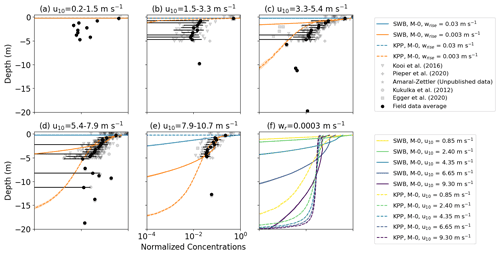Figure 2Vertical concentrations of buoyant particles for KPP and SWB diffusion using M-0 models. Panels (a)(e) show the vertical concentration profiles for high- and medium-buoyancy particles with increasing wind speeds. The KPP profiles are calculated for θ=1.0 and z0 according to Eq. (12). The grey markers indicate field measurements, with darker shades indicating more measurements, while the binned field measurement average and standard deviation are shown by the black markers. Panel (f) shows the vertical concentration profiles for low-buoyancy particles under increasing wind conditions. Shading around the profiles indicates the profile's standard deviation at each depth level.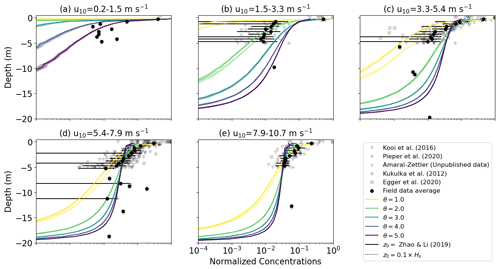Figure 3Vertical concentrations of buoyant particles for KPP diffusion using M-0 models for wr=0.003 m s−1. The KPP profiles are calculated for $\mathit{\theta }=\left[\mathrm{1.0},\mathrm{2.0},\mathrm{3.0},\mathrm{4.0},\mathrm{5.0}\right]$ and with either ${z}_{\mathrm{0}}=\mathrm{0.1}×{H}_{\mathrm{s}}$ or according to Eq. (12). The grey markers indicate field measurements, with darker shades indicating more measurements, while the binned field measurement average and standard deviation are shown by the black markers. Shading around the profiles indicates the profile's standard deviation at each depth level.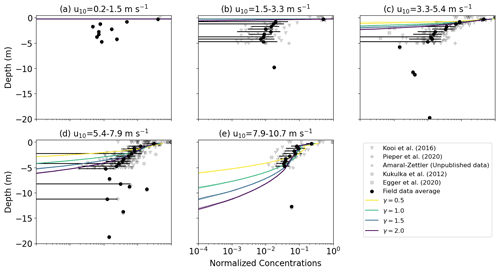Figure 4Vertical concentrations of buoyant particles for SWB diffusion under varying wind conditions with wr=0.003 m s−1. The SWB diffusion profile is calculated with $\mathit{\gamma }\in \left[\mathrm{0.5},\mathrm{1.0},\mathrm{1.5},\mathrm{2.0}\right]$. The grey markers indicate field measurements, with darker shades indicating more measurements, while the binned field measurement average and standard deviation are shown by the black markers. Shading around the profiles indicates the profile's standard deviation at each depth level.​​​​​​​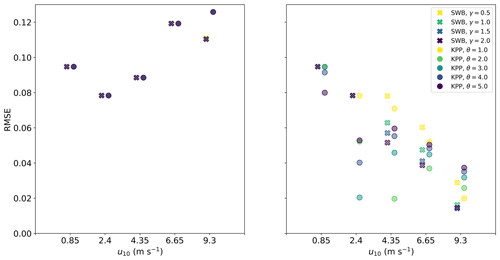Figure 5RMSE between field measurements and modeled concentration profiles for M-0 models with KPP and SWB diffusion under different wind conditions. All KPP diffusion simulations were with z0 according to Eq. (12).

With both KPP and SWB diffusion, M-1 models show deeper mixing of particles as α→1 (Fig. 6). Relative to the field measurements, M-1 models can at best slightly improve model performance over M-0 models (Fig. 7). However, improved model performance is not shown across all particle sizes and wind conditions, and there is not a consistent α value leading to the smallest RMSE values.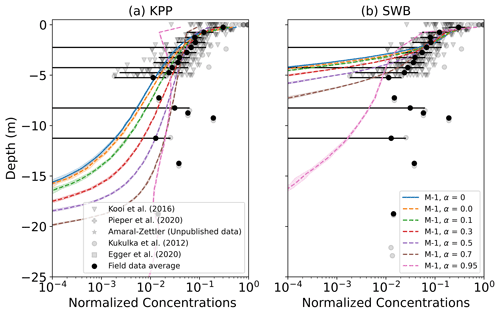Figure 6Vertical concentrations of buoyant particles for (a) KPP and (b) SWB diffusion using M-0 and M-1 models with varying values for α. The grey markers indicate field measurements, with darker shades indicating more measurements, while the binned field measurement average and standard deviation are shown by the black markers. Shading around the profiles indicates the profile's standard deviation at each depth level. The KPP profiles are for θ=1.0 and z0 according to Eq. (12). All profiles are for u10=6.65 m s−1 and medium-buoyancy particles (wrise=0.003 m s−1).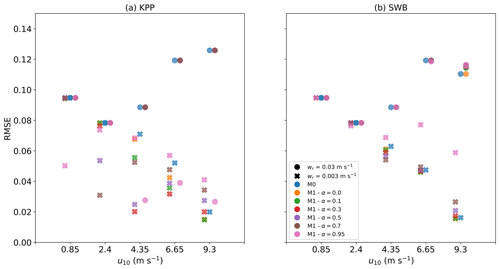Figure 7RMSE between field measurements and modeled concentration profiles for M-0 and M-1 models with (a) KPP and (b) SWB diffusion under different wind conditions and with varying values of α. All KPP diffusion simulations were with θ=1.0 and z0 according to Eq. 12.

4 Discussion

The parametrizations presented in this study are intended for use in 3D Lagrangian experiments using OGCM data and therefore should yield numerically stable results for the relatively large integration time steps used in large-scale Lagrangian vertical transport modeling . While there are more stable schemes available than the EM scheme used in this study , the EM scheme is computationally the cheapest and yields concentration profiles that match reasonably well with observations. Both M-0 and M-1 models show largely convergent concentration profiles for Δt=30 s, which would make both approaches feasible with regards to computational cost. However, we would currently recommend using a M-0 model. M-1 models have the additional tuning parameter α representing the autocorrelation of turbulent velocity fluctuations, which is poorly constrained in the literature. Using spatially invariant α values at best slightly improved model performance in comparison with M-0 models, and constraining α is not possible from these results. M-1 models may improve modeling of vertical diffusive transport, but more work is required to further constrain the value and vertical profile of α. Finally, numerous formulations of the M-1 drift term have been proposed (e.g., ) which can lead to large differences in the modeled profiles. In this study we used the non-normalized Langevin equation from , but other formulations could be explored in future work.

While the concentration profiles of medium- and low-buoyancy particles are unaffected by decreasing the integration time step Δt<30 s, using higher Δt values underestimates the depth to which high-buoyancy particles are mixed when using SWB diffusion. This is because for high Δt values, the upward non-stochastic component of Eq. (6), which scales with Δt, dominates the stochastic component, which scales with $\sqrt{\mathrm{\Delta }t}$. With KPP diffusion the vertical profile for high-buoyancy particles appears unaffected by Δt, but this is because the near-surface Kz values are significantly lower than with SWB diffusion. One possibility to correct for this is to apply a different BC, such as a reflective BC. While the concentration profiles for medium- and low-buoyancy particles are not strongly affected by such a reflective BC (Fig. G1), the reflective BC does show deeper particle mixing with SWB diffusion. However, for Δt=30 s the depth of mixing is now overestimated compared to smaller Δt values (Fig. G2), as with Δt=30 s and wr=0.03 m s−1 the particle would be reflected up to 0.9 m below the ocean surface solely due to the model numerics. In addition, earlier studies have shown that reflecting BC can cause spurious increases in particle concentration near the boundary . Therefore, changing the BC to a reflective BC would not improve the concentration profiles of high-buoyancy particles. Depending on the model application and setup, the error in the concentration profile depth (𝒪(1) m for high-buoyancy particles) might be acceptable. Otherwise, the error can be reduced by using a smaller integration time step where that is computationally feasible.

Considering the KPP and SWB diffusion profiles, the results in this study are inconclusive with regards to which approach performs better relative to field observations. For high-buoyancy particles, SWB diffusion leads to slightly deeper particle mixing, while only if the KPP diffusion profile accounts for LC-driven turbulence and has higher near-surface Kz values can it similarly show below-surface mixing of high-buoyancy particles for u10≥9.30 m s−1. With medium- and low-buoyancy particles the KPP profile leads to much deeper mixing compared with SWB diffusion where γ=1.0 , especially when accounting for LC-driven turbulence, and this appears to agree better with field observations. However, for SWB diffusion the value of γ is uncertain, as and define γ=1.0 and γ≈1.5, respectively. Higher γ values lead to approximately equal model performance relative to field observations as with KPP diffusion. However, the model evaluation is largely based on field measurements collected in the top 5 m of the water column, and it is below this depth that we see greater differences in the KPP and SWB vertical concentration profiles. In addition, the currently available data collected with neuston nets does not allow for model evaluation for the low-buoyancy particles in either scenario. As such, more field measurements (including smaller-sized particles) would be necessary to fully evaluate model performance for all particles sizes with the two diffusion profiles.

With regards to necessary data to calculate the diffusion profiles, the SWB approach has the benefit that it only requires surface wind stress data, while KPP diffusion additionally requires MLD data. In addition, while our results indicate that accounting for LC-driven turbulent mixing improves KPP diffusion model performance, determining which θ value to use is not trivial. demonstrated that θ is inversely proportional to the Langmuir number (La), which is defined as $\mathrm{La}=\sqrt{{u}_{*\mathrm{w}}/{U}_{\mathrm{S}}}$ with US as the surface Stokes drift. The Langmuir number can conceivably be calculated using OGCM data, but the details of such an implementation will be left for future work with 3D Lagrangian models. However, KPP diffusion does have the advantage that it has been widely used and validated in various model setups , while such extensive validation has not yet occurred for SWB diffusion. Finally, the influence of wind forcing on turbulence is generally assumed to be limited to the surface mixed layer , while with the SWB profile wind-generated turbulence can extend far below the MLD (Figs. 1 and E1), possibly overestimating turbulent mixing at such depths. KPP theory does limit wind-driven turbulent mixing to the surface mixed layer, while either a constant Kz value or other Kz profiles could be used for sub-MLD mixing, such as the Kz estimates for internal tide mixing as proposed by .

Ideally, KPP theory would be expanded to account for surface wave breaking, which could lead to higher near-surface Kz values as seen with MLD diffusion. While such a theoretical approach is beyond the scope of this paper, we show that artificially elevating near-surface Kz values by increasing the surface roughness z0 has a smaller influence on the overall concentration profile than LC-driven mixing, as similarly shown by . Therefore, although we recommend future work incorporating surface wave breaking into KPP theory, our current KPP diffusion approach representing LC-driving mixing through θ does already seem to capture the majority of turbulent mixing dynamics.

In all cases, the vertical concentration profiles stabilized to vertical equilibrium profiles, similar to what has been shown for buoyant particles in LES model studies . The modeled concentration profiles generally resembled the profiles from field measurements of microplastic concentrations under different wind conditions , but the averaged concentration profiles of the field measurements are quite noisy. This could be partly due to inhomogeneity in the particle buoyancy, as the collected microplastic particulates have varying sizes and rise velocities . Additionally, we sorted the field measurements based on wind conditions, but other underlying oceanographic conditions such as the MLD can still vary significantly even with similar wind speeds. Unfortunately, we lack additional data of the oceanographic conditions at the time of sampling, which currently prohibits more high-level comparisons of the field and model concentration profiles. Compared with the field data, the variance in the modeled concentration profiles is significantly smaller. This is in part also due to assuming constant environmental conditions over 12 h for the model simulations, while wind and other oceanographic conditions can change on much shorter timescales over the ocean surface. To further improve vertical transport model verification, more measurements would be required, covering a wider range of oceanographic conditions (such as for wind conditions higher than u10=10.7 m s−1) and with a high spatial sampling resolution also for depths $z<-\mathrm{5}$ m. Ideally these measurements would also sample small, neutrally buoyant particulates, but we acknowledge this is difficult with the sampling techniques commonly used today. At the same time, we would encourage conducting more ocean field measurements of near-surface vertical eddy diffusion coefficient and/or eddy viscosity profiles, as this will allow further validation of the Kz profiles predicted by the KPP and SWB theory with actual ocean near-surface mixing measurements.

The parameterizations have been validated for high and medium rise velocities, and at least for KPP diffusion with θ>1.0 the concentration profiles resemble those calculated from field observations. This provides confidence in the turbulence estimates from the KPP approach, and as these are independent of the type of particle that might be present, this would suggest the KPP approach can also be applied to neutral or negatively buoyant particles. However, as model verification was only possible for microplastic particulates with rise velocities between approximately 0.03 and 0.003 m s−1, we would advise additional model verification for other particle types where the necessary field data are available. In the case of SWB diffusion, turbulent mixing seems underestimated when further from the ocean surface when γ=1.0, but increasing to γ=1.5–2.0 does correct for this. However, as SWB diffusion has not yet been as extensively tested and verified as KPP diffusion, we advise more caution and additional validation with field observations before applying this diffusion approach to other particle types.

5 Conclusions

We have developed a number of 1D surface mixing parametrizations designed to be readily applied in large-scale oceanic Lagrangian model experiments using OGCM data. Where possible, we would recommend using the turbulence fields from the OGCM to assure turbulent transport of the particles is consistent with that of other model tracers. However, if the turbulence fields are unavailable then particularly parametrizations with KPP diffusion with LC-driven mixing are shown to produce modeled vertical concentration profiles that match relatively well with field observations of microplastics. The parametrizations generally perform well for time steps of Δt=30 s, but for high-buoyancy particles users need to take care to use sufficiently short time steps, especially with SWB diffusion. Verification was only possible for positively buoyant particles larger than 0.33 mm (which generally have rise velocities ≤0.003 m s−1), but the parametrizations should also be applicable to other particle types. The parametrizations can therefore be applied to investigate the influence of turbulent mixing on the vertical transport of (microplastic) particles within a 3D model setup, and ultimately gain a more complete understanding of the fate of such particles in the ocean.

Appendix A:${w}_{\mathrm{r}}/{w}^{\prime }$ ratios for various turbulence scenarios

Table A1Ratios ${w}_{\mathrm{r}}/{w}^{\prime }$ between the rise velocity wr and the peak stochastic velocity perturbation w for KPP and SWB diffusion. The peak w is the maximum value of Eq. (3). The peak w values for KPP diffusion are calculated for $\mathit{\theta }\in \left[\mathrm{1.0},\mathrm{3.0},\mathrm{5.0}\right]$ and for z0 following Eq. (12). The peak w values for SWB diffusion are independent of γ.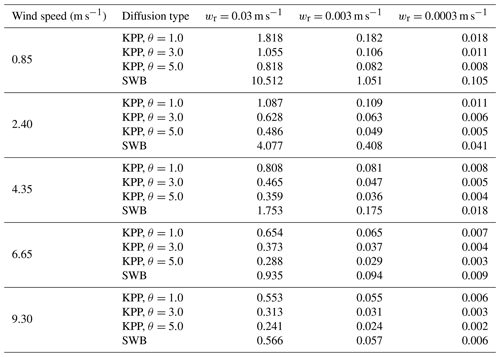Appendix B: Influence of z0 on diffusion profiles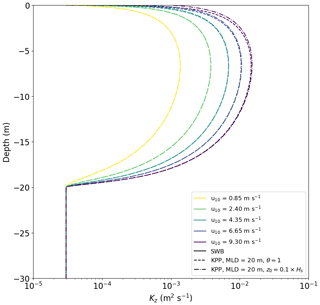Figure B1Vertical diffusion coefficient profiles for KPP diffusion under varying wind conditions with θ=1.0. The KPP diffusion profile is calculated with either z0 according to Eq. (12) or ${z}_{\mathrm{0}}=\mathrm{0.1}×{H}_{\mathrm{s}}$.

Appendix C: Time evolution of concentration profiles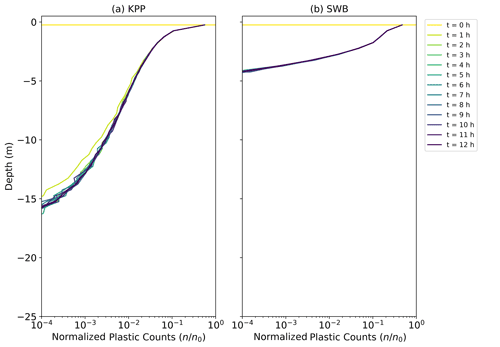Figure C1Vertical concentrations of buoyant particles for KPP diffusion at times t=0–12 h. The KPP diffusion profile is calculated with θ=1.0, u10=6.65 m s−1, and z0 according to Eq. (12).

Appendix D: Influence of θ for KPP diffusion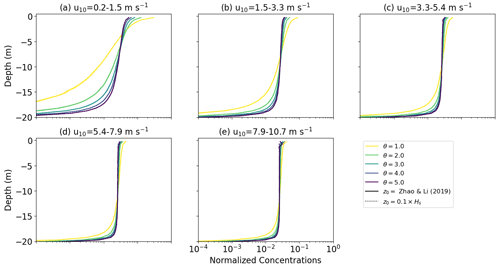Figure D1Vertical concentrations of buoyant particles for KPP diffusion under varying wind conditions with wr=0.0003 m s−1. The KPP diffusion profile is calculated with either z0 according to Eq. (12) or ${z}_{\mathrm{0}}=\mathrm{0.1}×{H}_{\mathrm{s}}$ and for $\mathit{\theta }\in \left[\mathrm{1.0},\mathrm{2.0},\mathrm{3.0},\mathrm{4.0},\mathrm{5.0}\right]$. The grey markers indicate field measurements, with darker shades indicating more measurements, while the binned field measurement average and standard deviation are shown by the black markers. Shading around the profiles indicates the profile's standard deviation at each depth level.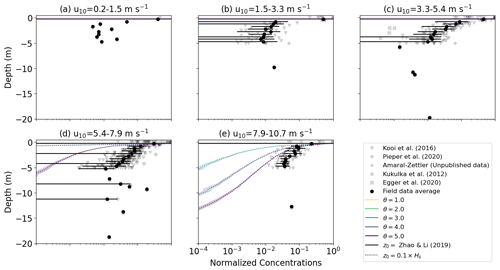Figure D2Vertical concentrations of buoyant particles for KPP diffusion under varying wind conditions with wr=0.03 m s−1. The KPP diffusion profile is calculated either with z0 according to Eq. (12) or ${z}_{\mathrm{0}}=\mathrm{0.1}×{H}_{\mathrm{s}}$ and for $\mathit{\theta }\in \left[\mathrm{1.0},\mathrm{2.0},\mathrm{3.0},\mathrm{4.0},\mathrm{5.0}\right]$. The grey markers indicate field measurements, with darker shades indicating more measurements, while the binned field measurement average and standard deviation are shown by the black markers. Shading around the profiles indicates the profile's standard deviation at each depth level.

Appendix E: Influence of γ for SWB diffusion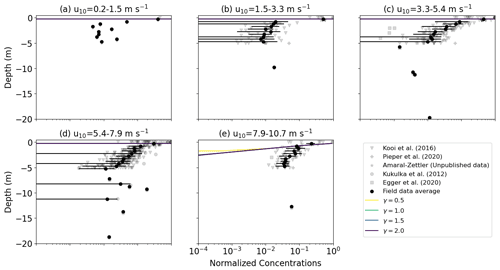Figure E1Vertical concentrations of buoyant particles for SWB diffusion under varying wind conditions with wr=0.0003 m s−1. The SWB diffusion profile is calculated with $\mathit{\gamma }\in \left[\mathrm{0.5},\mathrm{1.0},\mathrm{1.5},\mathrm{2.0}\right]$. The grey markers indicate field measurements, with darker shades indicating more measurements, while the binned field measurement average and standard deviation are shown by the black markers. Shading around the profiles indicates the profile's standard deviation at each depth level.Figure E2Vertical concentrations of buoyant particles for KPP diffusion under varying wind conditions with wr=0.03 m s−1. The SWB diffusion profile is calculated with $\mathit{\gamma }\in \left[\mathrm{0.5},\mathrm{1.0},\mathrm{1.5},\mathrm{2.0}\right]$. The grey markers indicate field measurements, with darker shades indicating more measurements, while the binned field measurement average and standard deviation are shown by the black markers. Shading around the profiles indicates the profile's standard deviation at each depth level.

Appendix F: Influence of Δt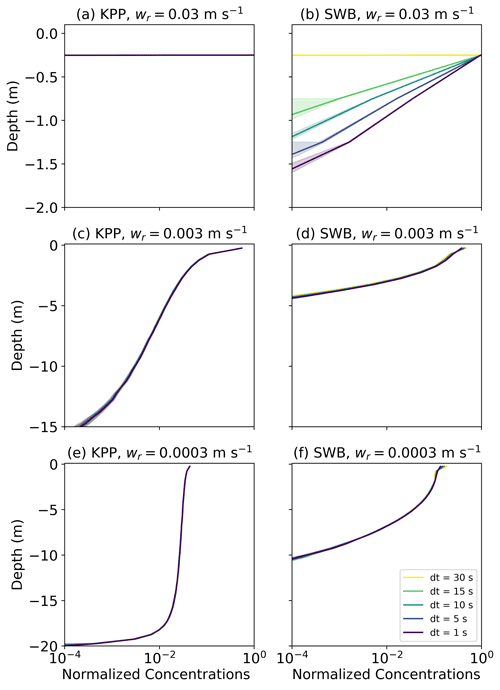Figure F1Vertical concentrations of buoyant particles for (a, c, e) KPP and (b, d, f) SWB diffusion using M-0 models with varying values for wrise and $\mathrm{\Delta }t\in \left[\mathrm{30},\mathrm{15},\mathrm{10},\mathrm{5},\mathrm{1}\right]$ s. All profiles are for u10=6.65 m s−1. Shading around the profiles indicates the profile's standard deviation at each depth level. The KPP profiles are computed with θ=1.0 and z0 according to Eq. (12), while the SWB profile is computed with γ=1.0.

Appendix G: Influence of boundary conditions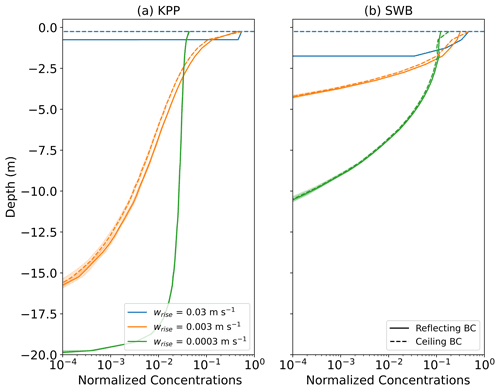Figure G1Vertical concentrations of buoyant particles for (a) KPP and (b) SWB diffusion using M-0 models for reflective and ceiling BCs. Shading around the profiles indicates the profile's standard deviation at each depth level. All profiles are for u10=6.65 m s−1. The KPP profiles are computed with θ=1.0 and z0 according to Eq. (12), while the SWB profile is computed with γ=1.0.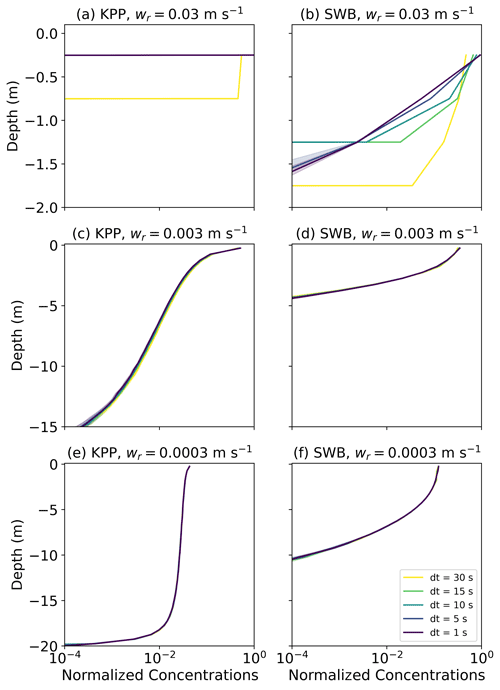Figure G2Vertical concentrations of buoyant particles for (a, c, e) KPP and (b, d, f) SWB diffusion using M-0 models with varying values for wrise and $\mathrm{\Delta }t\in \left[\mathrm{30},\mathrm{15},\mathrm{10},\mathrm{5},\mathrm{1}\right]$ s with a reflective BC. All profiles are for u10=6.65 m s−1. Shading around the profiles indicates the profile's standard deviation at each depth level. The KPP profiles are computed with θ=1.0 and z0 according to Eq. (12), while the SWB profile is computed with γ=1.0.

Code and data availability

The code for the 1D model, the subsequent analysis and all figures are available from Zenodo (https://doi.org/10.5281/zenodo.5886711, ). The field data for are available from figshare (https://doi.org/10.6084/m9.figshare.3427862.v1, ). For the field data from , , and Amaral-Zettler (unpublished data), please contact the corresponding authors of the respective studies.

Author contributions

Development of the parametrizations and the analysis was done by VO, with CL helping with improving the code performance. The manuscript was written by VO, with extensive input from CL and EvS. Everyone contributed to the study design and discussion of the analysis.

Competing interests

The contact author has declared that neither they nor their co-authors have any competing interests.

Disclaimer

Publisher's note: Copernicus Publications remains neutral with regard to jurisdictional claims in published maps and institutional affiliations.

Acknowledgements

Calculations were performed on UBELIX (http://www.id.unibe.ch/hpc, last access: 21 January 2022), the HPC cluster at the University of Bern. We would like to thank Tobias Kukulka, Catharina Pieper, Matthias Egger, Linda Amaral-Zettler, and Erik Zettler for providing field measurements; Marie Poulain-Zarcos for providing data on vertical mixing; and Thomas Stocker​​​​​​​ and Daan Reijnders for fruitful discussions regarding the Markov-1 numerical schemes.

Financial support

This research has been supported by the Schweizerischer Nationalfonds zur Förderung der Wissenschaftlichen Forschung (grant no. PZ00P2_174124) and the European Research Council, H2020 European Research Council (TOPIOS grant no. 715386).

Review statement

This paper was edited by David Ham and reviewed by Matthieu Mercier and one anonymous referee.

References

Berloff, P. S. and McWilliams, J. C.: Material transport in oceanic gyres. Part III: Randomized stochastic models, J. Phys. Oceanogr., 33, 1416–1445, 2003. a, b, c

Boufadel, M., Liu, R., Zhao, L., Lu, Y., Özgökmen, T., Nedwed, T., and Lee, K.: Transport of oil droplets in the upper ocean: impact of the eddy diffusivity, J. Geophys. Res.-Oceans, 125, e2019JC015727, https://doi.org/10.1029/2019JC015727, 2020. a, b, c

Brickman, D. and Smith, P.: Lagrangian stochastic modeling in coastal oceanography, J. Atmos. Ocean. Tech., 19, 83–99, 2002. a, b, c, d, e

Brignac, K. C., Jung, M. R., King, C., Royer, S.-J., Blickley, L., Lamson, M. R., Potemra, J. T., and Lynch, J. M.: Marine debris polymers on main Hawaiian Island beaches, sea surface, and seafloor, Environ. Sci. Technol., 53, 12218–12226, 2019. a

Brunner, K., Kukulka, T., Proskurowski, G., and Law, K. L.: Passive buoyant tracers in the ocean surface boundary layer: 2. Observations and simulations of microplastic marine debris, J. Geophys. Res.-Oceans, 120, 7559–7573, 2015. a, b, c, d

Chamecki, M., Chor, T., Yang, D., and Meneveau, C.: Material transport in the ocean mixed layer: recent developments enabled by large eddy simulations, Rev. Geophys., 57, 1338–1371, 2019. a, b, c

Choy, C. A., Robison, B. H., Gagne, T. O., Erwin, B., Firl, E., Halden, R. U., Hamilton, J. A., Katija, K., Lisin, S. E., Rolsky, C., and Van Houtan, K. S.​​​​​​​: The vertical distribution and biological transport of marine microplastics across the epipelagic and mesopelagic water column, Scientific Reports, 9, 7843​​​​​​​, https://doi.org/10.1038/s41598-019-44117-2, 2019. a

Craig, P. D. and Banner, M. L.: Modeling wave-enhanced turbulence in the ocean surface layer, J. Phys. Oceanogr., 24, 2546–2559, 1994. a

de Boyer Montégut, C., Madec, G., Fischer, A. S., Lazar, A., and Iudicone, D.: Mixed layer depth over the global ocean: An examination of profile data and a profile-based climatology, J. Geophys. Res.-Oceans, 109, C12003, https://doi.org/10.1029/2004JC002378, 2004. a

Delandmeter, P. and van Sebille, E.: The Parcels v2.0 Lagrangian framework: new field interpolation schemes, Geosci. Model Dev., 12, 3571–3584, https://doi.org/10.5194/gmd-12-3571-2019, 2019. a

de Lavergne, C., Vic, C., Madec, G., Roquet, F., Waterhouse, A. F., Whalen, C., Cuypers, Y., Bouruet-Aubertot, P., Ferron, B., and Hibiya, T.: A parameterization of local and remote tidal mixing, J. Adv. Model. Earth Sy., 12, e2020MS002065, https://doi.org/10.1029/2020MS002065, 2020. a

Denman, K. and Gargett, A.: Time and space scales of vertical mixing and advection of phytoplankton in the upper ocean, Limnol. Oceanogr., 28, 801–815, 1983. a, b

Egger, M., Sulu-Gambari, F., and Lebreton, L.: First evidence of plastic fallout from the North Pacific Garbage Patch, Scientific Reports, 10, 7495​​​​​​​, https://doi.org/10.1038/s41598-020-64465-8, 2020. a, b, c, d, e, f

Enders, K., Lenz, R., Stedmon, C. A., and Nielsen, T. G.: Abundance, size and polymer composition of marine microplastics ≥10µm in the Atlantic Ocean and their modelled vertical distribution, Mar. Pollut. Bull., 100, 70–81, 2015. a

Fernando, H. J.: Turbulent mixing in stratified fluids, Annu. Rev. Fluid Mech., 23, 455–493, 1991. a

Fischer, R., Lobelle, D., Kooi, M., Koelmans, A., Onink, V., Laufkötter, C., Amaral-Zettler, L., Yool, A., and van Sebille, E.: Modeling submerged biofouled microplastics and their vertical trajectories, Biogeosciences Discuss. [preprint], https://doi.org/10.5194/bg-2021-236, in review, 2021. a

Gaspar, P., Grégoris, Y., and Lefevre, J.-M.: A simple eddy kinetic energy model for simulations of the oceanic vertical mixing: Tests at station Papa and Long-Term Upper Ocean Study site, J. Geophys. Res.-Oceans, 95, 16179–16193, 1990. a

Gräwe, U., Deleersnijder, E., Shah, S. H. A. M., and Heemink, A. W.: Why the Euler scheme in particle tracking is not enough: the shallow-sea pycnocline test case, Ocean Dynam., 62, 501–514, 2012. a, b, c

Hopfinger, E. and Toly, J.-A.: Spatially decaying turbulence and its relation to mixing across density interfaces, J. Fluid Mech., 78, 155–175, 1976. a

Kaiser, D., Kowalski, N., and Waniek, J. J.: Effects of biofouling on the sinking behavior of microplastics, Environ. Res. Lett., 12, 124003, https://doi.org/10.1088/1748-9326/aa8e8b, 2017. a

Kooi, M., Reisser, J., Slat, B., Ferrari, F., Schmid, M., Cunsolo, S., Brambini, R., Noble, K., Sirks, L.-A., Linders, T. E., Schoeneich-Argent, R. I., and Koelmans, A. A.: Data from “The effect of particle properties on the depth profile of buoyant plastics in the ocean”, FigShare [data set], https://doi.org/10.6084/m9.figshare.3427862.v1, 2016a. a

Kooi, M., Reisser, J., Slat, B., Ferrari, F. F., Schmid, M. S., Cunsolo, S., Brambini, R., Noble, K., Sirks, L.-A., Linders, T. E. W., Schoeneich-Argent, R. I., and Koelmans, A. A.​​​​​​​: The effect of particle properties on the depth profile of buoyant plastics in the ocean, Scientific Reports, 6, 33882, https://doi.org/10.1038/srep33882, 2016b. a, b, c, d, e, f, g, h, i, j

Kukulka, T. and Brunner, K.: Passive buoyant tracers in the ocean surface boundary layer: 1. Influence of equilibrium wind-waves on vertical distributions, J. Geophys. Res.-Oceans, 120, 3837–3858, 2015. a

Kukulka, T. and Veron, F.: Lagrangian investigation of wave-driven turbulence in the ocean surface boundary layer, J. Phys. Oceanogr., 49, 409–429, 2019. a, b

Kukulka, T., Proskurowski, G., Morét-Ferguson, S., Meyer, D., and Law, K.: The effect of wind mixing on the vertical distribution of buoyant plastic debris, Geophys. Res. Lett., 39, L07601, https://doi.org/10.1029/2012GL051116, 2012. a, b, c, d, e, f, g, h, i, j, k, l, m, n, o, p, q, r

Landahl, M. T. and Christensen, E. M.: Turbulence and random processes in fluid mechanics, 2nd edn., Cambridge University Press, ISBN10 0521422132, ISBN-13 978-0521422130, 1992. a

Large, W. and Pond, S.: Open ocean momentum flux measurements in moderate to strong winds, J. Phys. Oceanogr., 11, 324–336, 1981. a

Large, W. G., McWilliams, J. C., and Doney, S. C.: Oceanic vertical mixing: A review and a model with a nonlocal boundary layer parameterization, Rev. Geophys., 32, 363–403, 1994. a, b, c, d

Law, K. L., Morét-Ferguson, S. E., Goodwin, D. S., Zettler, E. R., DeForce, E., Kukulka, T., and Proskurowski, G.: Distribution of surface plastic debris in the eastern Pacific Ocean from an 11-year data set, Environ. Sci. Technol., 48, 4732–4738, 2014. a

Lebreton, L., Slat, B., Ferrari, F., Sainte-Rose, B., Aitken, J., Marthouse, R., Hajbane, S., Cunsolo, S., Schwarz, A., Levivier, A., Noble, K., Debeljak, P., Maral, H., Schoeneich-Argent, R., Brambini, R., and Reisser, J.​​​​​​​: Evidence that the Great Pacific Garbage Patch is rapidly accumulating plastic, Scientific Reports, 8, 4666​​​​​​​, https://doi.org/10.1038/s41598-018-22939-w, 2018. a

Liang, J.-H., McWilliams, J. C., Sullivan, P. P., and Baschek, B.: Large eddy simulation of the bubbly ocean: New insights on subsurface bubble distribution and bubble-mediated gas transfer, J. Geophys. Res.-Oceans, 117, C04002, https://doi.org/10.1029/2011JC007766, 2012. a, b

Liubartseva, S., Coppini, G., Lecci, R., and Clementi, E.: Tracking plastics in the Mediterranean: 2D Lagrangian model, Mar. Pollut. Bull., 129, 151–162, 2018. a

Lobelle, D., Kooi, M., Koelmans, A. A., Laufkötter, C., Jongedijk, C. E., Kehl, C., and van Sebille, E.: Global modeled sinking characteristics of biofouled microplastic, J. Geophys. Res.-Oceans, 126, e2020JC017098, https://doi.org/10.1029/2020JC017098, 2021. a, b, c

Maruyama, G.: Continuous Markov processes and stochastic equations, Rendiconti del Circolo Matematico di Palermo, 4, 48–90​​​​​​​, https://doi.org/10.1007/BF02846028, 1955. a

McWilliams, J. C. and Sullivan, P. P.: Vertical mixing by Langmuir circulations, Spill Sci. Technol. B., 6, 225–237, 2000. a, b, c

Mofakham, A. A. and Ahmadi, G.: On random walk models for simulation of particle-laden turbulent flows, Int. J. Multiphas. Flow, 122, 103157, https://doi.org/10.1016/j.ijmultiphaseflow.2019.103157, 2020. a, b, c

Nordam, T., Kristiansen, R., Nepstad, R., and Röhrs, J.: Numerical analysis of boundary conditions in a Lagrangian particle model for vertical mixing, transport and surfacing of buoyant particles in the water column, Ocean Model., 136, 107–119, 2019. a

Onink, V.: Model and analysis code for: “Empirical Lagrangian parametrization for wind-driven mixing of buoyant particulates at the ocean surface”, Zenodo [code], https://doi.org/10.5281/zenodo.5886711, 2021. a

Onink, V., Wichmann, D., Delandmeter, P., and van Sebille, E.: The role of Ekman currents, geostrophy, and stokes drift in the accumulation of floating microplastic, J. Geophys. Res.-Oceans, 124, 1474–1490, 2019. a, b, c

Onink, V., Jongedijk, C. E., Hoffman, M. J., van Sebille, E., and Laufkötter, C.: Global simulations of marine plastic transport show plastic trapping in coastal zones, Environ. Res. Lett., 16, 064053, https://doi.org/10.1088/1748-9326/abecbd, 2021. a, b

Paris, C. B., Atema, J., Irisson, J.-O., Kingsford, M., Gerlach, G., and Guigand, C. M.: Reef odor: a wake up call for navigation in reef fish larvae, PloS one, 8, e72808, https://doi.org/10.1371/journal.pone.0072808, 2013. a

Pieper, C., Martins, A., Zettler, E., Loureiro, C. M., Onink, V., Heikkilä, A., Epinoux, A., Edson, E., Donnarumma, V., de Vogel, F., Law, K. L., and Amaral-Zettler, L.​​​​​​​: INTO THE MED: Searching for Microplastics from Space to Deep-Sea, in: International Conference on Microplastic Pollution in the Mediterranean Sea, edited by: Di Pace, M. C. E, Errico, M. E., Gentile, G., Montarsolo, A., Mossotti, R., and Avella, M., Springer, 129–138, https://doi.org/10.1007/978-3-030-45909-3_21, 2019. a, b, c, d, e, f

Poulain, M.: Etude de la distribution verticale de particules plastiques dans l'océan: caractérisation, modélisation et comparaison avec des observations, PhD thesis, Institut National Polytechnique de Toulouse, 6 allée Emile Monso – BP 34038 31029, Toulouse, https://oatao.univ-toulouse.fr/27525/ (last access: 21 January 2022​​​​​​​), 2020. a, b, c, d, e, f

Poulain, M., Mercier, M. J., Brach, L., Martignac, M., Routaboul, C., Perez, E., Desjean, M. C., and Ter Halle, A.: Small microplastics as a main contributor to plastic mass balance in the North Atlantic Subtropical Gyre, Environ. Sci. Technol., 53, 1157–1164, 2019. a

Riisgård, H. U. and Larsen, P. S.: Viscosity of seawater controls beat frequency of water-pumping cilia and filtration rate of mussels Mytilus edulis, Mar. Ecol. Prog. Ser., 343, 141–150, 2007. a

Ross, O. N. and Sharples, J.: Recipe for 1-D Lagrangian particle tracking models in space-varying diffusivity, Limnol. Oceanogr.-Meth., 2, 289–302, 2004. a, b

Samaras, A. G., De Dominicis, M., Archetti, R., Lamberti, A., and Pinardi, N.: Towards improving the representation of beaching in oil spill models: A case study, Mar. Pollut. Bull., 88, 91–101, 2014. a

Taylor, J. R.: Accumulation and subduction of buoyant material at submesoscale fronts, J. Phys. Oceanogr., 48, 1233–1241, 2018. a, b, c

Thompson, S. and Turner, J.: Mixing across an interface due to turbulence generated by an oscillating grid, J. Fluid Mech., 67, 349–368, 1975. a

Van Sebille, E., Griffies, S. M., Abernathey, R., Adams, T. P., Berloff, P., Biastoch, A., Blanke, B., Chassignet, E. P., Cheng, Y., Cotter, C. J., Deleersnijder, E., Döös, K., Drake, H. F., Drijfhout, S., Gary, S. F., Heemink, A. W., Kjellsson, J., Koszalka, I. M., Lange, M., Lique, C., MacGilchrist, G. A., Marsh, R., Mayorga Adame, C. G., McAdam, R., Nencioli, F., Paris, C. B., Piggott, M. D., Polton, J. A., Rühs, S., Shah, S. H. A. M., Thomas, M. D., Wang, J., Wolfram, P. J., Zanna, L., and Zika, J. D.​​​​​​​: Lagrangian ocean analysis: Fundamentals and practices, Ocean Model., 121, 49–75, 2018. a, b

Van Sebille, E., Aliani, S., Law, K. L., Maximenko, N., Alsina, J. M., Bagaev, A., Bergmann, M., Chapron, B., Chubarenko, I., Cózar, A., Delandmeter, P., Egger, M., Fox-Kemper, B., Garaba, S. P., Goddijn-Murphy, L., Hardesty, B. D., Hoffman, M. J., Isobe, A., Jongedijk, C. E., Kaandorp, M. L. A., Khatmullina, L., Koelmans, A. A., Kukulka, T., Laufkötter, C., Lebreton, L., Lobelle, D., Maes, C., Martinez-Vicente, V., Morales Maqueda, M. A., Poulain-Zarcos, M., Rodríguez, E., Ryan, P. G., Shanks, A. L., Shim, W. J., Suaria, G., Thiel, M., van den Bremer, T. S., and Wichmann, D.: The physical oceanography of the transport of floating marine debris, Environ. Res. Lett., 15, 023003, https://doi.org/10.1088/1748-9326/ab6d7d, 2020.  a

Waterhouse, A. F., MacKinnon, J. A., Nash, J. D., Alford, M. H., Kunze, E., Simmons, H. L., Polzin, K. L., St. Laurent, L. C., Sun, O. M., Pinkel, R., Talley, L. D., Whalen, C. B., Huussen, T. N., Carter, G. S., Fer, I., Waterman, S., Naveira Garabato, A. C., Sanford, T. B., and Lee, C. M.: Global patterns of diapycnal mixing from measurements of the turbulent dissipation rate, J. Phys. Oceanogr., 44, 1854–1872, 2014. a

Wichmann, D., Delandmeter, P., and van Sebille, E.: Influence of near-surface currents on the global dispersal of marine microplastic, J. Geophys. Res.-Oceans, 124, 6086–6096, 2019. a

Yang, D., Chamecki, M., and Meneveau, C.: Inhibition of oil plume dilution in Langmuir ocean circulation, Geophys. Res. Lett., 41, 1632–1638, 2014. a, b

Zhao, D. and Li, M.: Dependence of wind stress across an air–sea interface on wave states, J. Oceanogr., 75, 207–223, 2019. a, b, c